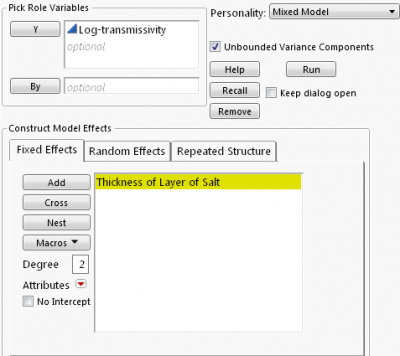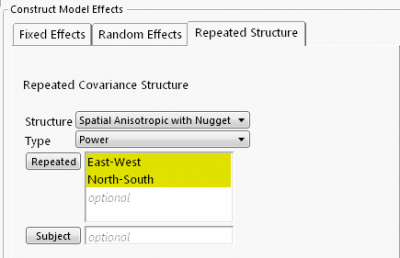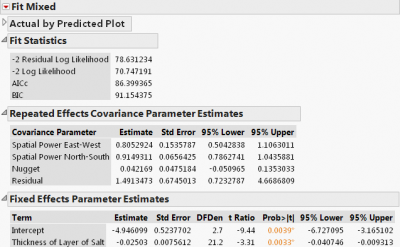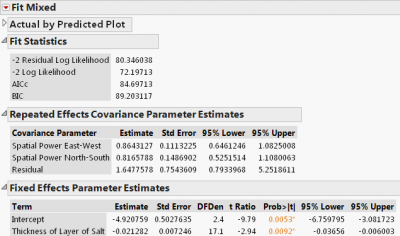Choose Language Hide Translation Bar
JMP Pro for linear mixed models — Part 3

This is the final post in my JMP for Linear Mixed Models series (see my earlier posts: Part 1 and Part 2). Here, I will show an example of spatial regression, followed by some tips for fitting mixed models in JMP Pro.

Example 4: Modeling geospatial data — taking spatial correlation into account

Just as repeated measures are correlated over time spatial data are likely correlated in space — that is, measurements that are relatively close together are more alike than those farther apart. Thus, we need to take spatial dependency into account in the analysis.

Spatial data are recorded along with coordinates such as latitude and longitude, positions of row and column, north-south and east-west directions. The distance between two measurements is calculated using a Euclidean distance function, which is used to form a covariance structure. If a distance function doesn't depend on the directions of measurements, then the covariance is said to be isotropic; otherwise, it is anisotropic. In addition, a nugget effect can be added to account for abrupt changes over small distances in a local area.

JMP Pro provides four Euclidean distance functions for isotropic structures: power, exponential, Gaussian and spherical. Various forms of anisotropic structures are available. A nugget effect can also be added to covariance structures.

The following example is taken from SAS for Mixed Models (2nd Edition, 2006, pp. 457-460). In order to investigate the water drainage at a hazardous waste disposal site, 30 samples were taken at various locations at the site and recorded by their north-south and east-west directions. A linear relationship between water drainage (measured by log-transmissivity) and the thickness of a layer of salt was proposed. (The data,  Data Set 11.6, Hazardous Waste,  is available in a zip file from SAS.)

I fit the following spatial regression model using a spatial anisotropic power structure with a nugget effect. This structure allows (1) distance to be a power function of spatial correlation, (2) spatial correlations to differ in different directions and (3) variation over small distances.Fixed effects part of the modelSpatial anisotropic power with nugget

In the Repeated Effects Covariance Parameter Estimate, two spatial correlation estimates, 0.81 (East-West) and 0.91 (North-South), are highly significant. However, the estimated nugget effect is not statistically significant. Fixed Effects Parameter Estimates show a significant negative effect on water drainage of thickness of salt.Results using spatial anisotropic power with nugget

I refit the model without a nugget effect.Results using spatial anisotropic power without nugget

We notice some minor changes in the covariance and fixed effect estimates: The estimated spatial correlations are 0.86 and 0.82, respectively, and the effect of thickness of salt is -0.021. Comparing the goodness of fit using AICc, the second model is slightly better as its AICs is smaller (84.7 vs. 86.4).

To formally test the existence of spatial correlation, we fit an independent errors model by selecting Residual as the structure (i.e., assuming no spatial correlation). The difference in -2 Residual Log Likelihood value between two models forms a χ2 likelihood ratio test. The -2 Residual Log Likelihood from the independent errors model (not shown here) is 94.07, so the difference is 13.72 (=94.07-80.35). This yields a p-value of 0.001 for DF=2. Therefore, significant spatial correlation is found at this site.

Conclusion and tips

By now, I hope I have demonstrated the versatility of mixed models and ease of fitting a mixed model in JMP. Before I close, I’d like to offer some general tips:

• In order to run a mixed model, data needs to be organized in a “tall and skinny” format where multiple measures of a response are stacked into a single column. If your data is in a “short and wide” format, use the JMP Tables function Stack to transpose.
• Follow the JMP Repeated Covariance Structure Requirements when entering Repeated and Subject columns.
• Try different covariance structures and evaluate different models by comparing AICc or BIC. The smaller the AICc (or BIC), the better the fit of a model. Ceteris paribus, a parsimonious model is better.
• An independent errors model (i.e., a model with fixed effects only and a residual repeated structure) can serve as a baseline model for performing a χ2 likelihood ratio test on the existence of a covariance  structure as shown above.
• When both random effects and repeated effects are include in a model, there is often insufficient data to estimate both effects. For example, JMP doesn't allow random effects when an unstructured covariance is selected.
• Finally, I will be presenting a live webcast, Linear Mixed Models Using JMP Pro, on July 17 at 2 p.m. EDT. You are welcome to attend.

(Acknowledgement: I would like to thank Christopher Gotwalt and Laura Lancaster for their help.)

Article Labels
Article Tags